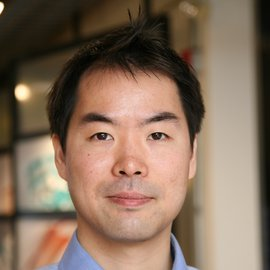# A Curvature and Density‐based Generative Representation of Shapes### Abstract

This paper introduces a generative model for 3D surfaces based on a representation of shapes with mean curvature and metric, which are invariant under rigid transformation. Hence, compared with existing 3D machine learning frameworks, our model substantially reduces the influence of translation and rotation. In addition, the local structure of shapes will be more precisely captured, since the curvature is explicitly encoded in our model. Specifically, every surface is first conformally mapped to a canonical domain, such as a unit disk or a unit sphere. Then, it is represented by two functions":" the mean curvature half‐density and the vertex density, over this canonical domain. Assuming that input shapes follow a certain distribution in a latent space, we use the variational autoencoder to learn the latent space representation. After the learning, we can generate variations of shapes by randomly sampling the distribution in the latent space. Surfaces with triangular meshes can be reconstructed from the generated data by applying isotropic remeshing and spin transformation, which is given by Dirac equation. We demonstrate the effectiveness of our model on datasets of man‐made and biological shapes and compare the results with other methods.

Publication
Computer Graphics Forum 40(1) 2021